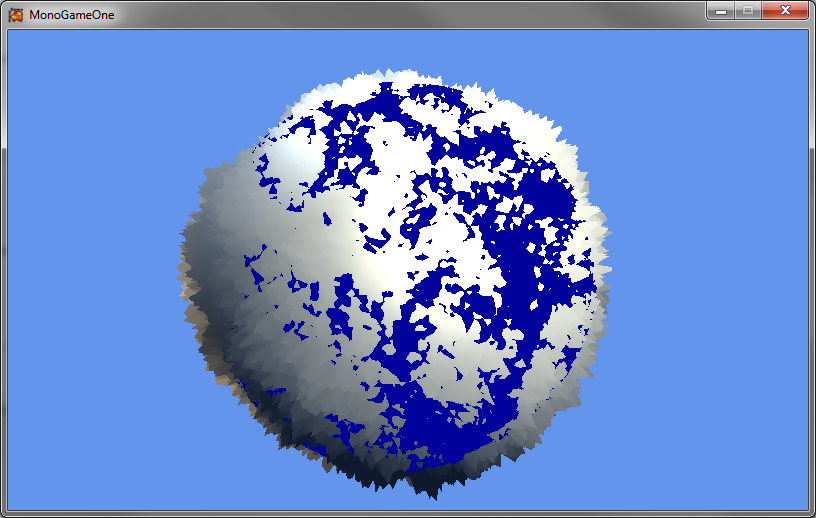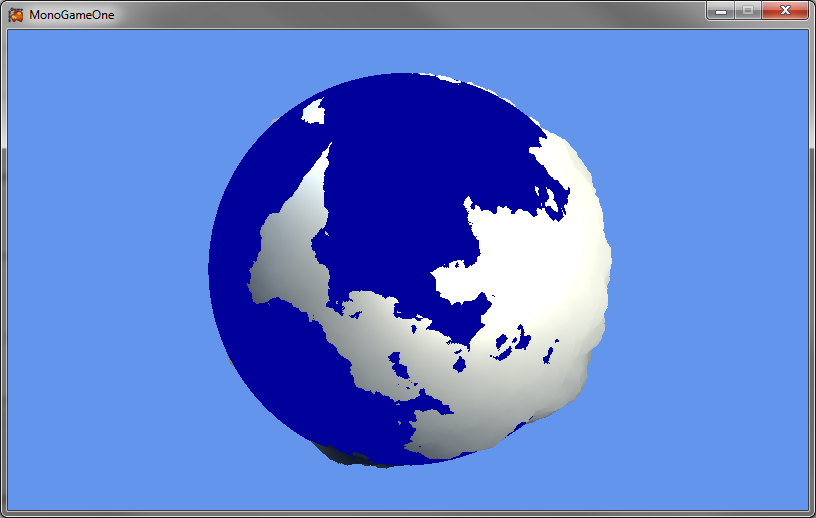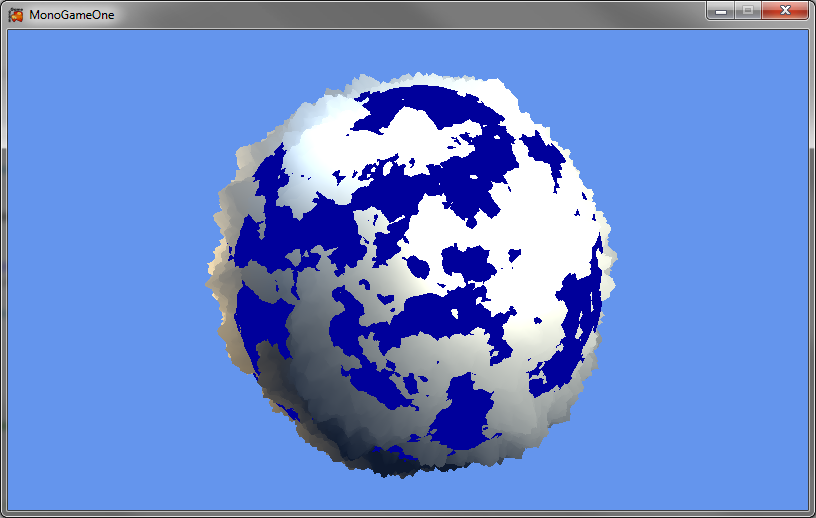Feb 12 2013

# Generating Planets – Part Two – Terrain

So, in the previous post we generated our sphere, and in this post we are going to turn our smooth sphere into a planet with terrain. I am going to assume you already have a basic understanding of how fractal terrain generation works on a square grid, but if not you should first read this article.

With our subdivided icosahedron the terrain generation works in very much the same way, each time we are generating a new vertex we average it between the two parent vertices and then assign it a random modifier of its own. Depending on how exactly you implemented your algorithm this may require a number of different steps, but in this post I will describe it as if you are using a similar set up to the code linked in my previous post.

Before we start adding random numbers to our vertices we are going to need to add in some way to be able to find the height of the two parent nodes, this step wouldn’t be necessary on a simple square grid, but the fact we have already needed to modify the height of our vertex to put it on the edge of the sphere complicates things slightly.

My solution is to add a second list alongside the list of vertices (named the vertexList in the example code). This list will store the various heights we have generated for our vertices. This is actually pretty easy to implement, all you need to do is when sending the 3D position, p, to the method for adding vertices to the list is to also send the two heights with it, which can be pulled from the height list in the same way the 3D positions of the parent nodes are. Then, in the method that adds the vertices, you average these two heights, add your own random component, and store it in the height list.

At this point, the planet would look something like this:A nice random mess of spikes, but not exactly a planet.

The problem is that you need to reduce the amount of noise each time you subdivide the planet in order to make it refine the heights rather than just turning them into a spikey mess. Again, this is simple to do, you just add a counter to the iteration part of your algorithm that makes the number of the current iteration available to the method that adds the vertices, and then use that to reduce the size of the random number you are adding. I suggest using something like:

` noise = (((float)random.NextDouble() - 0.5f) * structure.noise * ((float)Math.Pow(0.5, 1)));`

Where structure.noise is a preset variable that decides how “noisy” the terrain is. This would give you a planet that looks a lot nicer, a bit like this:Its starting to look a lot better, but there is still one major problem. There will always tend to be one large continent and one large ocean. This is because there are only twenty vertices on our base icosahedron that have the maximum amount of noise given to them. This is the equivalent problem to having too few points on our starting square based grid when generating a traditional heightmap. Again, this is pretty simple to solve, we simply turn off the part that uses averages to determine our vertices height, and the part that reduces the magnitude of the random addition until a set iteration. This will allow us to have smaller continents and a more archipelago style map (my personal favorite map type for RTS’s and Civ games).

This leaves us with a planet that looks like below, or alternatively, with correct modification of the variables, a large number of different and unique planets.The code I use for this is:

```float noise = 0; if (currentlevelofsubdivision &lt; structure.noiseclamp) { noise = (((float)random.NextDouble() - 0.5f) * structure.noise * ((float)Math.Pow(structure.noiseconstant, 1))); } else { noise = (parentOneHeight + parentTwoHeight) / 2; noise += (((float)random.NextDouble() - 0.5f) * structure.noise * ((float)Math.Pow(structure.noiseconstant, currentlevelofsubdivision - structure.noiseclamp))); } Vector3 n = Vector3.Normalize(p) * noise; heightList.Add(noise);```

Where structure.noiseclamp is the predetermined subdivision iteration at which it starts reducing the magnitude of the added noise and starts averaging between the heights of the two parent nodes.